### Real Number System Class 9th Mathematics Term 1 Tamilnadu Board Solution

##### Question 1.A number having non-terminating and recurring decimal expansion is –A. an integerB. a rational numberC. an irrational numberD. a whole numberAnswer:By definition, a rational number is one which follows either of the following 2 conditions:1. It can be expressed in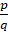form where p and q are integers (q≠0).2. Its decimal expansion is either terminating or non-terminating recurring.Ex. – 0.35 (terminating decimal expansion) → Rational number0.7777…. =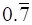(non-terminating but recurring i.e. the number does not terminate but its digits follow a pattern.) → Rational numberπ = 3.14…… (Non-terminating non recurring) → Irrational numberHence, the answer.Question 2.If a number has a non-terminating non-recurring decimal expansion then the number is –A. a rational numberB. a natural numberC. an irrational numberD. an integer.Answer:As explained in the above question, for a number to be rational its decimal expansion has to be either terminating or non-terminating and recurring.For example –π = 3.14…… (Non-terminating non recurring) → Irrational numberHence, the answer.Question 3.Decimal form of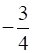isA. −0.75B. −0.50C. −0.25D. −0.125Answer:To convert into its equivalent decimal expansion, we can either proceed by long division method or multiply the denominator and (hence) numerator by a number such that the denominator turns out to be 100.Now, let 4×a = 100Solving, we get a = 25.Hence multiplying numerator and denominator by 25.∴ (-3/4) × (25/25) = (-75/100) = -0.75 (Placing decimal point before 2 digits).Hence, the answer.Question 4.The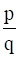form of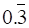isA.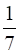B.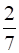C.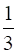D.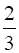Answer:Let x = 0.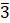= 0.3333... → (eq)1Since there is only one repeating digit after decimal point, multiply equation 1 by 10.10x = 3.3333… = 3 + 0.3333… = 3 + x → (eq)2(eq)2 –(eq)110x-x = 3.3333… - 0.3333… = 3∴ 9x = 3∴ x = 1/3Hence, the answer.Question 5.Which one of the following is not true?A. Every natural number is a rational numberB. Every real number is a rational numberC. Every whole number is a rational numberD. Every integer is a rational number.Answer:Real numbers is the set which includes both rational and irrational numbers.∴ Rational numbers and Irrational numbers are 2 independent subsets of Real numbers. Hence every real number is not a rational number (since real numbers also consists of irrational numbers.)Option (A) is correct because every natural number can be expressed inform (q≠0 i.e a rational number).Option (C) is correct because every whole number can be expressed inform (q≠0 i.e a rational number).Option (D) is correct because every integer can be expressed inform (q≠0 i.e a rational number).Natural numbers ⊂ Whole numbers ⊂ Integers ⊂ Rational Numbers ⊂ Real numbersReal numbers ⊃ (Rational numbers + Irrational numbers).Hence, only option B is incorrect.Question 6.Which one of the following has a terminating decimal expansion?A.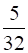B.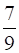C.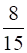D.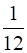Answer:If a rational number(q≠0) can be expressed in the form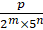, where p ∈ Z, and m,n ∈ W, then the rational number will have a terminating decimal expansion.Otherwise, the rational number will have a non-terminating and recurring decimalexpansion.Now, 32 = 25 × 50 Hence, it has terminating decimal expansion.Hence, option A is correct.Option B is incorrect ∵ 9 = 32 and it cannot be expressed in the form 2m × 5n.Option C is incorrect ∵ 15 = 3 × 5 and it cannot be expressed in the form 2m × 5n.Option D is incorrect ∵ 12 = 3 × 22 and it cannot be expressed in the form 2m × 5n.Hence, only option A is correct.Question 7.Which one of the following is an irrational number?A. πB.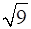C.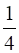D.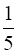Answer:Option A is correct since π = 3.141592… i.e. the decimal expansion is non-terminating and non-recurring.Option B is incorrect because= ±3 and it is an integer (and hence a rational number, Integers ⊂ Rational Numbers).Option C is incorrect as it is expressed in the form(p = 1 and q = 4) and is a rational number (by definition, q≠0).Option D is incorrect as it is expressed in the form(p = 1 and q = 5) and is a rational number (by definition, q≠0).Hence, option A is correct.Question 8.Which of the following are irrational numbers?i.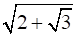ii.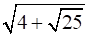iii.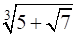iv.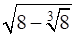A. (ii), (iii) and (iv)B. (i), (ii) and (iv)C. (i), (ii) and (iii)D. (i), (iii) and (iv)Answer:i.√(2 + √3 ) is irrational ∵ √3 is irrational and 2 is rational and sum of a rational and irrational number is always irrational. Hence 2 + √3 is irrational. Hence, its square root is also irrational.ii.√(4 + √25) is rational ∵ √25( = 5) is rational and 4 is also rational.Hence, √(4 + 5) = √9 = 3 is rational.iii. ∛(5 + √7) is irrational ∵ √7 is irrational and 5 is rational and sum of a rational and irrational number is always irrational. Hence 5 + √7 is irrational. Hence, its cube root is also irrational.iv.√(8-∛8 ) is irrational. Here both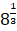( = 2) and 8 are rational. Hence8 – 81/3 = 8 – 2 = 6 is rational. But √6 is irrational.Hence, the answer.

PDF FILE TO YOUR EMAIL IMMEDIATELY PURCHASE NOTES & PAPER SOLUTION. @ Rs. 50/- each (GST extra)

HINDI ENTIRE PAPER SOLUTION

MARATHI PAPER SOLUTION

SSC MATHS I PAPER SOLUTION

SSC MATHS II PAPER SOLUTION

SSC SCIENCE I PAPER SOLUTION

SSC SCIENCE II PAPER SOLUTION

SSC ENGLISH PAPER SOLUTION

SSC & HSC ENGLISH WRITING SKILL

HSC ACCOUNTS NOTES

HSC OCM NOTES

HSC ECONOMICS NOTES

HSC SECRETARIAL PRACTICE NOTES

2019 Board Paper Solution

HSC ENGLISH SET A 2019 21st February, 2019

HSC ENGLISH SET B 2019 21st February, 2019

HSC ENGLISH SET C 2019 21st February, 2019

HSC ENGLISH SET D 2019 21st February, 2019

SECRETARIAL PRACTICE (S.P) 2019 25th February, 2019

HSC XII PHYSICS 2019 25th February, 2019

CHEMISTRY XII HSC SOLUTION 27th, February, 2019

OCM PAPER SOLUTION 2019 27th, February, 2019

HSC MATHS PAPER SOLUTION COMMERCE, 2nd March, 2019

HSC MATHS PAPER SOLUTION SCIENCE 2nd, March, 2019

SSC ENGLISH STD 10 5TH MARCH, 2019.

HSC XII ACCOUNTS 2019 6th March, 2019

HSC XII BIOLOGY 2019 6TH March, 2019

HSC XII ECONOMICS 9Th March 2019

SSC Maths I March 2019 Solution 10th Standard11th, March, 2019

SSC MATHS II MARCH 2019 SOLUTION 10TH STD.13th March, 2019

SSC SCIENCE I MARCH 2019 SOLUTION 10TH STD. 15th March, 2019.

SSC SCIENCE II MARCH 2019 SOLUTION 10TH STD. 18th March, 2019.

SSC SOCIAL SCIENCE I MARCH 2019 SOLUTION20th March, 2019

SSC SOCIAL SCIENCE II MARCH 2019 SOLUTION, 22nd March, 2019

XII CBSE - BOARD - MARCH - 2019 ENGLISH - QP + SOLUTIONS, 2nd March, 2019

HSC Maharashtra Board Papers 2020

(Std 12th English Medium)

HSC ECONOMICS MARCH 2020

HSC OCM MARCH 2020

HSC ACCOUNTS MARCH 2020

HSC S.P. MARCH 2020

HSC ENGLISH MARCH 2020

HSC HINDI MARCH 2020

HSC MARATHI MARCH 2020

HSC MATHS MARCH 2020

SSC Maharashtra Board Papers 2020

(Std 10th English Medium)

English MARCH 2020

HindI MARCH 2020

Hindi (Composite) MARCH 2020

Marathi MARCH 2020

Mathematics (Paper 1) MARCH 2020

Mathematics (Paper 2) MARCH 2020

Sanskrit MARCH 2020

Sanskrit (Composite) MARCH 2020

Science (Paper 1) MARCH 2020

Science (Paper 2)

MUST REMEMBER THINGS on the day of Exam

Are you prepared? for English Grammar in Board Exam.

Paper Presentation In Board Exam

How to Score Good Marks in SSC Board Exams

Tips To Score More Than 90% Marks In 12th Board Exam

How to write English exams?

How to prepare for board exam when less time is left

How to memorise what you learn for board exam

No. 1 Simple Hack, you can try out, in preparing for Board Exam

How to Study for CBSE Class 10 Board Exams Subject Wise Tips?

JEE Main 2020 Registration Process – Exam Pattern & Important Dates

NEET UG 2020 Registration Process Exam Pattern & Important Dates

How can One Prepare for two Competitive Exams at the same time?

8 Proven Tips to Handle Anxiety before Exams!